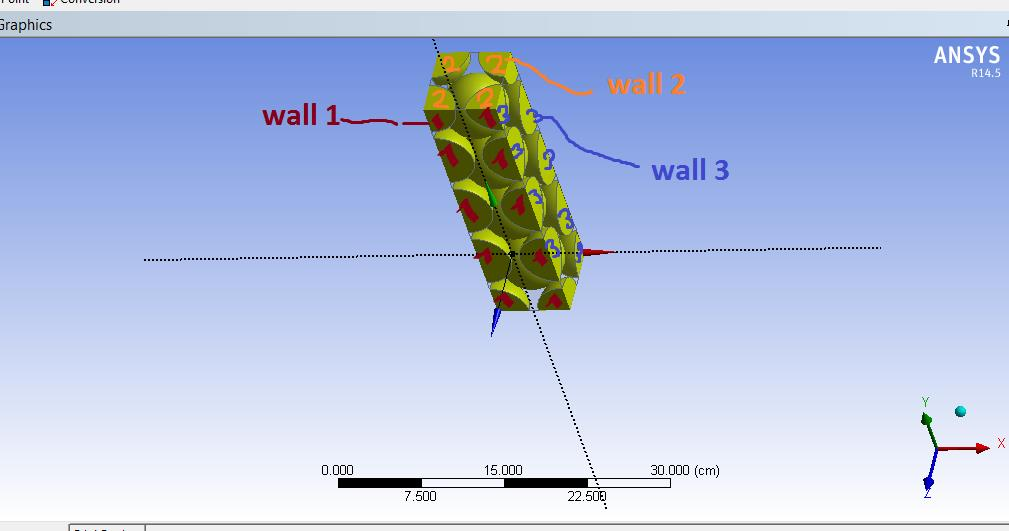## Fluids

•hamda
Subscriber

Hi there,

In my problem, I have defined 2 domains; solid domain (spheres) and fluid domain (the space between them).
In sub domain in solid domain  I have defined heat source. Indeed each sphere is a fuel element. Now I have a problem with boundary condition in solid domain (fuel domain).  As you can see in the following picture there are several spheres (fuel elements)  and in boundary conditions in Heat Transfer section, I don't know which option is the best  to my problem. I selected "heat transfer coefficient " but there is a problem to define "coefficient" and  outside temperature.  I have the value of power density so I can calculate the energy for each sphere and also for all spheres. My problem is in order to calculate "heat transfer coefficient" should I consider all spheres? or I should consider each sphere and calculate its thermal resistance?•DrAmine
Ansys Employee

Does it look if you have totally submerged spheres in your fluids but some fractions of sphere pointing to the outer? The walls of the outer can be set to Robin B.C with ambient temperature and HTC from standard engineering book if the spheres are suspended in air (5-20 W/m^2K). But I think that you have in reality spheres submerged in your moderator and that you are simplifying the geometry: is this true? What about applying symmetry or periodic conditions? What about building up the simplified model with only fluid outer walls bounding a certain number of spheres? Perhaps other community member might chime in here.

•hamda
Subscriber

This is a slice of a packed bed reactor. The spheres are fuel elements and a coolant gas like helium flows through them and collects heat from them.

And yes, some fractions of spheres pointing to the outer. and so I don;t consider them as a complete sphere and so the fuel volume would be  8 times the volume of sphere.

I want to apply symmetry boundary to for   six sides.  I hope there is no problem when I want to run. But  Now my problem is in this section:

I created a subdomain in fuel domain to introduce a energy source and then I realized that in the fuel domain and also coolant domain I should specify the type of  Heat transfer. I thought it was not reasonable to select adiabatic or fixed T.